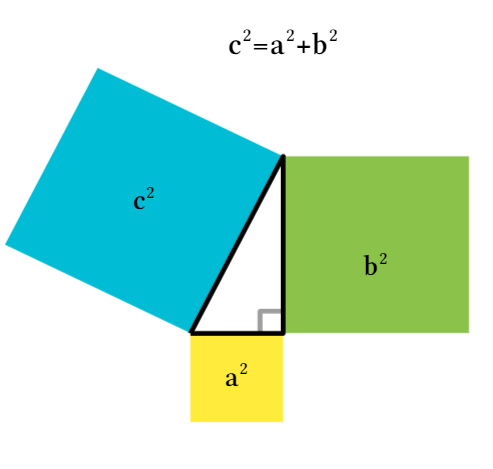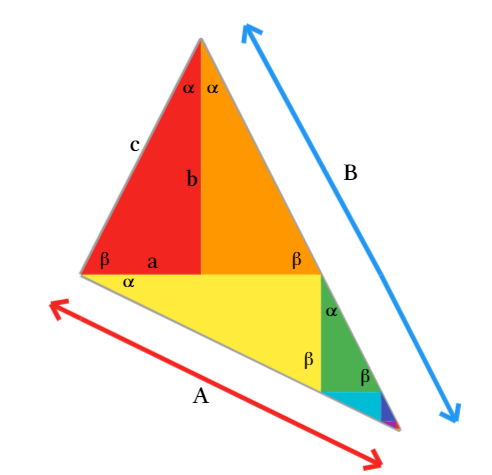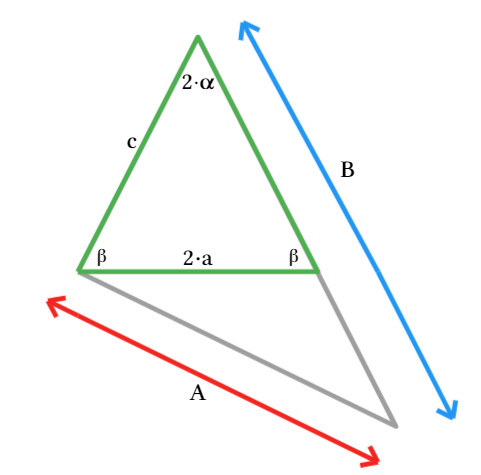## New Proof of The Pythagorean Theorem!

Maple Learn

In March of 2023, two high school students, Calcea Johnson, and Ne’Kiya Jackson, presented a new proof of the Pythagorean Theorem at the American Mathematical Society’s Annual Spring Southeastern Sectional Meeting. These two young women are challenging the conventions of math as we know it.
The Pythagorean Theorem states that in a right angle triangle, the sum of the squares of the legs is equal to the square of the hypotenuse:The theorem has been around for over two thousand years and has been proven hundreds of times with many different methods. So what makes the Johnson-Jackson proof special? The proof is one of the first to use trigonometry.
For years, mathematicians have been convinced that a trigonometric proof of the Pythagorean Theorem is impossible because much of trigonometry is based upon the Pythagorean Theorem itself (an example of circular reasoning).
That said, some results in trigonometry are independent of the Pythagorean Theorem, namely the law of sines, and the sine and cosine ratios; the latter is a result that 12-year-old Einstein used in his trigonometric proof of the theorem.
Though all the details of the Johnson-Jackson proof have not been made public, there was enough information for me to recreate the proof in Maple Learn. The idea of the proof is to construct a right angle triangle with an infinite series of congruent right angle triangles (the first of which has side lengths a, b, and c). Then, using the sine ratio, solve for the hypotenuse lengths of each small congruent triangle. To explore this construction see Johnson and Jackson’s Triangle Construction on Maple Learn.Next, find the side lengths of the large triangle (A and B) by evaluating an infinite sum (composed of the hypotenuse lengths of the small congruent triangles). Finally, apply the law of sines to the isosceles triangle made from the first 2 congruent triangles. After simplifying this expression, the Pythagorean relationship (c2 = a2 + b2) emerges.To see more details of the proof, check out Johnson and Jackson’s Proof of Pythagorean Theorem on Maple Learn.
This new proof of the Pythagorean Theorem shows that discoveries in math are still happening and that young people can play a big role in these discoveries!﻿